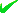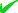Country(s)

Industry News

October 2019
TuMoSuSaFrThWe
1514131211109

New Math K-Plus Features: Number Powers, Exponents, and Roots

Newly added features include lessons in Number Powers, Exponents, and Roots
By: Private Company
MARLTON, N.J. - Aug. 14, 2015 - PRLog -- Math K-Plus works to build children’s arithmetic problem solving skills. Lesson resources address the needs of children from kindergarten through sixth grade. Topics covered in details: numbers, counting, addition, subtraction, multiplication, division, fractions, math properties, number factoring, and numerical expressions. All resources operate at the pace of individual student. New resources fall into the following categories.

1) Power lessons cover raising any number to a power, multiplication, division, of powers, etc.

2) Exponent lessons cover how to multiply, and divide, numbers with exponents, etc.

3) Roots include product properties, quotient properties, and simplification of square roots.

4) Each subject has calculators so students can verify their homework, but more importantly can experiment and learn by practice.

Email Tags : ***@gmail.com: K-12, Math, Powers, Exponents, Roots, Multiplication, Division, Calculators : Education : Marlton - New Jersey - United States
Account Email AddressAccount Phone NumberDisclaimer     Report Abuse